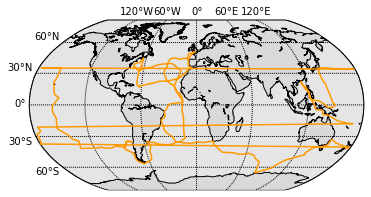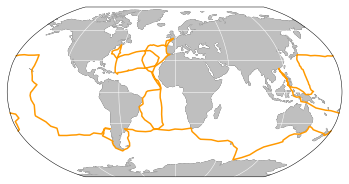# Cartopy as an alternative to basemap

The previous post I've mentioned both iris and cartopy modules, and talked a little bit on the use of iris to download, read and plot a gridded data-set (A.K.A. the iris cube). Here I'll show a little bit more about cartopy.

The best feature so far is that cartopy fixes one of the most annoying bugs in basemap, the handling of datelines. Here is an example:

Let's start by loading the Challenger Expedition track and define a few common properties for plotting with both basemap and cartopy.

In :
import numpy as np
import matplotlib.pyplot as plt

kw = dict(color='#FF9900', linestyle='-', linewidth=1.5)
lon, lat = np.loadtxt('./data/challenger_path.csv', delimiter=',', unpack=True)


We can easily define a function to create a Basemap figure with the robinson projection.

In :
from mpl_toolkits.basemap import Basemap

def make_basemap(projection='robin', figsize=(6, 4), resolution='c'):
fig, ax = plt.subplots(figsize=figsize)
m = Basemap(projection=projection, resolution=resolution,
lon_0=0, ax=ax)
m.drawcoastlines()
m.fillcontinents(color='0.85')
parallels = np.arange(-60, 90, 30.)
meridians = np.arange(-360, 360, 60.)
m.drawparallels(parallels, labels=[1, 0, 0, 0])
m.drawmeridians(meridians, labels=[0, 0, 1, 0])
return fig, m

fig, m = make_basemap()
_ = m.plot(*m(lon, lat), **kw)The problem with datelines is evident, instead of wrapping "behind" the figure, basemap connects the lines when we cross the dateline. There are some ways to work around this issue, but they might involve changing your map in ways you do not wish to do. Now let's try the same map with cartopy.

The block below defines a function to create our figure in a very similar fashion as we did for Basemap.

In :
import cartopy.crs as ccrs
import cartopy.feature as cfeature

def make_cartopy(projection=ccrs.Robinson(), figsize=(6, 4), resolution='110m'):
fig, ax = plt.subplots(figsize=figsize, subplot_kw=dict(projection=projection))
ax.set_global()
ax.coastlines(resolution=resolution, color='k')
# Only PlateCarree and Mercator plots are currently supported.
gl = ax.gridlines(draw_labels=False)
return fig, ax

fig, ax = make_cartopy(projection=ccrs.Robinson(), resolution='110m')
_ = ax.plot(lon, lat, transform=ccrs.Geodetic(), **kw)

/home/filipe/miniconda3/envs/Blog/lib/python2.7/site-packages/matplotlib/artist.py:221: MatplotlibDeprecationWarning: This has been deprecated in mpl 1.5, please use the
axes property.  A removal date has not been set.
warnings.warn(_get_axes_msg, mplDeprecation, stacklevel=1)Much better!

In :
HTML(html)

Out:

This post was written as an IPython notebook. It is available for download or as a static html.